## Introduction

In a synchronized state, the units of a system are in the same (or similar) dynamical states at every time1,2. The interest of scientists in understanding the relationship between the emergence of such a collective behavior and the physical mechanisms governing the transfer and processing of information in a system of networking units, motivated the first studies of synchronization in oscillator networks with complex topologies3,4. Since then, synchronization phenomena and complex networks have been extensively studied hand in hand, highlighting the crucial role of the network topology in the emergence and stability of the synchronous motion5,6,7,8,9,10,11,12. For instance, it has been found that synchronization can emerge more easily in networks with highly heterogeneous degree distribution, due to the presence of nodes sharing a large number of connections (the hubs) that may act as pacemakers for the rest of the oscillators5,13,14. On the other hand, the presence of hubs reduces considerably the stability of fully synchronized states15. In addition to this, since densely interconnected sets of oscillators synchronize more easily than those with sparse connections16,17, the analysis of synchronization has also been used as a tool to detect the presence of modules at different topological scales6,18,19.

All the works mentioned above consider synchronization on static complex networks. However, fixed interaction patterns turn out to be inadequate for the description of many real-world networks, which are intrinsically time-varying20,21,22,23. More importantly, the stationary hypothesis must be abandoned when modeling the situations where the very same network topology emerges as a result of the dynamical interaction between its constituents. For these reasons, the interest has moved to adaptive networks24, i.e., to graphs where topology co-evolves with the dynamical process taking place on top of them, thus creating a feedback loop between structure and dynamics.

Such an interplay between structure and dynamics is a rather general principle that spans different contexts, ranging from interpersonal relationships in society to neuronal networks in the brain. In these two latter settings, the ties between connected elements are known to be strongly favored by the similarity of their dynamical states, the latter being described as individual opinions or firing rates. This similarity-driven interaction principle is nowadays widely accepted in both sociology and neuroscience under the terms of homophily25 and Hebbian learning26, respectively. The homophily principle is, for instance, at the core of the internal mechanisms governing the transfer and processing of information at the level of individual and, at higher scales, it plays a leading role in the development of cultural consensus27 in society and cognitive tasks in the brain28. In both cases, the latter macroscopic behaviors can be described as the emergence of a synchronized state in which individuals start to behave in a coherent way.

Models considering the effects of synchronization on the structure of a network have been recently proposed29,30,31,32. In these works, the ties between units (or groups of them) strengthen as their dynamical states become more and more similar. However, in order to describe the structure and dynamics of real systems, such as social or neural ones, we need to add a further constraint to the reinforcing mechanism acting on each single link. In fact, it is reasonable to introduce the effects of homeostasis, i.e. a competition mechanism by which the enhancement of some connection from a node is counter-balanced by the weakening of other connections of the same node to the network. In practice, this second ingredient considers that the available resources devoted to sustain interactions, i.e. the local wiring around each node of the network, are finite. Once again, this competition mechanism is observed in real social and neural systems. In the former case, the time invested for establishing social relationships is always limited, thus imposing a careful choice of the acquaintances. Moreover, the value of the number of social relationships that a person is able to sustain in a stable way is widely known as Dunbar’s number33. On the other hand, in neural systems, competition appears in combination with Hebbian learning in the development of nerve connections as an essential ingredient of their physiological plasticity34,35.

We here introduce a simple model of an adaptive network of phase oscillators, in which both mechanisms of homophily and homeostasis are taken into account. The dynamics of the oscillators is regulated by the Kuramoto model36,37 which is a paradigmatic framework for the study of synchronization processes in many systems38,39. We show that the feedback mechanism provided by the combination of homophily and homeostasis leads to the emergence of structural and dynamical features observed in many real systems, such as scale-free distribution of interaction weights, strong modularity and a striking enhancement of local synchronization in the absence of global dynamic order.

We consider a weighted and directed network of N coupled phase-oscillators, where the phase of the i-th unit (i = 1, …, N) is denoted by θi (t) and evolves in time according to:where ωi stands for the natural frequency of i, λ is the coupling constant and WijWij (t) are non-negative quantities representing the strength at time t of the links pointing from nodes j to i. The specific case in which the weights Wij are the same for all pairs of nodes i and j and do not depend on time, was introduced by Kuramoto as a simple model to describe how a synchronized state (a state in which θi (t) = θj (t) i, j and t) emerges in a system of interacting dynamical units for large enough values of λ 36,40,41.

In our model, the weights of the interactions Wij (t) in equation (1) co-evolve with the system dynamics as:where si is the total incoming strength of node i,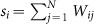and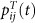is the degree of local synchronization between oscillators i and j, averaged over time in the interval [tT, t]13,14: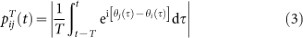In the above equations, T is a control parameter that quantifies the amount of memory used by each oscillator in the updating process and the quantities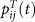take values in [0, 1], with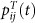meaning that oscillators i and j have been perfectly synchronized along the last T time units.

The adaptive scheme in equation (2) has the form of the replicator equation of evolutionary dynamics42 and is inspired to the need of retaining the main characteristics of both homophily and homeostasis. The inputs j which in the last T time units have been highly synchronized with the target node i (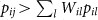), will enhance their strength, according to homophily. On the other hand, as a consequence of homeostasis, the weights of the remaining inputs (those with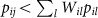) will be depressed to keep constant the total incoming strength, si , of oscillator i, that is initially set equal to si = 1 i. In this way, homeostasis naturally arises from equation (2) by checking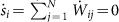. As a consequence, all the links pointing to the same oscillator compete for the available resources.

## Results

In the top panels of Fig. 1 we report the typical time-evolution of the order parameter r for different values of the two control parameters λ and T. For t < 0, we integrated numerically equation (1) on a homogeneous network in which all the nodes have k neighbors and the weights Wij do not change in time and are fixed to 1/k for all links. Then, for t ≥ 0 we considered the full dynamics of the adaptive model by switching on the weights’ evolution governed by equation (2). When the weights co-evolve with the oscillators’ dynamics, a clear enhancement of synchronization is observed for any values of λ and T. However, in some cases, as in those panels corresponding to λ = 0.50 and 0.65, the order parameter r exhibits quasi-periodic oscillations in time.

The different phases of the oscillators’ dynamics in the λ − T plane are characterized by the contour-plots in Fig. 1. The diagrams report the values of the degree of global, r (left) and local, rlink (right), synchrony obtained in the stationary state of the dynamics (see the Methods section). By looking at the contour-plot of the global order parameter, r, we can identify three different dynamical regimes, whose boundaries are highlighted by dashed curves. In particular, going from small to large values of λ we move from an incoherent phase (phase I) to a totally synchronized region (phase III), passing by a partially ordered phase (phase II). On the other hand, the contourplot of rlink describes the local degree of synchronization within the network. When the system is ordered at a global scale, both partially or totally, it also attains a perfect local synchronization. Conversely, different areas in the region of global incoherence correspond to different local regimes. We will now discuss in detail each of the three phases.

We start from phase III which, as shown in the contour plots of Fig. 1, appears for relatively large values of the coupling strength λ. In this phase the dynamics of the system ends up in a perfectly synchronized state (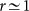) and the resulting network is very similar to the initial network i.e., a regular structure in which all the links share roughly the same value of the weights. This result indicates that, for values of λ close to the critical point of the non-adaptive network, the adaptive system simply needs a weak reorganization of the weights to achieve full synchrony. However, this perfect synchronization is different from what observed in real scenarios: full opinion consensus and complete neural synchronization are quite unusual in social and neural settings. Therefore, in the remainder of this paper we will rather focus in describing the emergent behavior in the dynamical regimes where a local synchronization appears while a perfect global dynamical order is absent, i.e. in phases I and II of the parameter space.

We turn first our attention to phase II. Here, the system exhibits partial global order, with a value of r(t) oscillating in time, together with an almost perfect local degree of synchronization. In Fig. 2 (a) we report a typical case in which the order parameter r(t) behaves harmonically. A careful analysis of the network structure points out that, as an effect of the adaptive dynamics, a large fraction of the links have been suppressed, i.e. are left with a weight which is practically zero. This behavior is very different from what we have observed found in phase III, where all the links survive, slightly modifying their original weights. Here the network splits into two clusters, practically two separate components, which are clearly visualized by plotting the weight matrix, after an opportune relabeling of the nodes. Each of the two components displays a large degree of internal synchrony. In fact, if we evaluate r(t) separately for each of the two clusters, we find in both cases a value of r which is close to 1 and constant in time. Hence, the clusters can be regarded as two almost non-interacting oscillators with different natural frequencies ω 1, ω 2. The interference between such frequencies produces the harmonic behavior of r(t) with frequency Ω1,2 = ω 1 − ω 2. In Fig. 2 (b) we also report a case in which the network splits into three components, each one exhibiting an almost perfect degree of internal synchronization. Again, the modules can be regarded as three independent oscillators with natural frequencies ω 1, ω 2, ω 3 and, as expected, the order parameter r(t) of the whole network oscillates periodically with leading frequencies Ω1,2 = ω 1 − ω 2, Ω1,3 = ω 1 − ω 3 and Ω2,3 = ω 2 − ω 3. The spontaneous break up of the initial network into separate components is the typical situation we have found for all values of λ and T in phase II. However, the partitions can be far more complex than the two cases described above and multiple components of different sizes can coexist in the asymptotic network state. Moreover, we have also observed that a further community structure may appear inside one of the components of the network (as we will later show in Fig. 3) pointing out a highly nontrivial modular structure.

To better characterize the modularity of the networks produced, we have computed the modular cohesion, MC, of the resulting partition (see the Methods section). As shown in Fig. 2 (c), the MC takes its maximal value, MC = 1, in phase II, indicating a partition of the network into separated frequency components. Notice that the value of MC remains rather large also in phase I, where the network still displays a modular structure, while some weak links connecting different modules appear, thus making the overall network connected. The appearance of different connected modules in phase I reveals the emergence of meso-scale features from the dynamical reorganization of the weights. Modular structures frequently appear in large social and neural systems. In particular, in the brain of mammals, they efficiently describe the organization of the cortico-cortical pathways into anatomical-functional modules43,44. In a social context, modular patterns are identified with those densely interconnected groups of individuals sharing similar opinions and cultural interests. In phase I of our model, a modular structure emerges spontaneously altogether with other key features empirically found in large scale cortical networks45. Namely, for large values of T the internal synchrony of each module is remarkably large, while the global order parameter is very close to zero, as indicated by rlink and r shown in Fig. 1. Even more striking, the network structure obtained in phase I displays scale-free architectures for the local connectivity patterns. In particular, in Fig. 3 we report the distribution of link weights, P(Wij ), obtained at T = 100 for different values of λ. For low values of λ (corresponding to phase I and the beginning of phase II), our model produces a hierarchical distribution of weights at all scales, which can be fitted by a power-law distribution,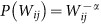, with an exponent α ranging in [0.85, 1.2]. These scalefree architectures coexist (see the network snapshots in the right part of Fig. 3 corresponding to λ = 0.1 and 0.2) with a highly modular architecture in which networks are composed of several communities of different sizes. The different modules are connected by small weight links, while internal links have strong weights. As λ increases (see λ = 0.3 and 0.4), the intra-modules links increase their weight, as can be observed from the increase in the peak of P(Wij ) at large values of Wij . The enhancement of intra-module ties occurs at the expense of the weakening of the weights of inter-module links and the eventual break up of the network into several components with independent dynamical behaviors. However, as can be observed from the network snapshots, each of the components may contain several modules of smaller sizes, thus leading to networks with hierarchical modular behavior. Finally, when phase III approaches (see λ = 0.5 and 0.6), the topology of the network turns to be more compact and link weights tend to be rather homogeneous.

## Discussion

The emergence of highly modular structures altogether with scale-free interaction patterns in our adaptive network model reproduces, respectively at the mesoscopic and microscopic level, two universal properties of real networks. In addition to this, the two structural properties occur when the system displays a large degree of local synchronization in the absence of global dynamical order. Our findings are thus in agreement with either dynamical and structural features observed in real neural and social systems. In such systems, on one hand, local synchronization and consensus coexist with a lack of global order while, on the other hand, modularity and scale-free interaction patterns are core features of their backbone. In particular, regarding the scaling of edge weights, recent quantitative studies on the wiring of fibers in large cortical brain networks have reported power-law distributions for axon fiber densities46,47 in agreement with those displayed by our adaptive network model. Concerning social systems, the recent development of large scale online social networks and the burst of data about social communications through mobile networks is allowing to monitor the degree of friendship between connected users through the analysis of the load of communication between connected users. Recent research in this direction points out that the load of information in this one-to-one communication channels follows a power-law distribution48,49,50 corroborating again the scale-free patterns of the interaction weights.

Summing up, in the present work we have introduced and analyzed a simple model of an adaptive network of oscillators, where the evolution of the topology is regulated by the synchronization dynamics through the competing mechanisms of homophily and homeostasis. The adaptive nature of the interactions produces a better synchronization both at global and local scale with respect to the non-adaptive case. At the same time, the link weights evolve towards non-trivial stationary states. The model presents three main phases as a function of its control parameters. In the first phase, an enhancement of global and local synchronization is achieved by a coordinated finetuning of the link weights. In the second phase, partial global order is attained together with an almost perfect degree of local synchronization. In practice, the network spontaneously splits into a number of components, each formed by perfectly synchronized oscillators. In particular, the model produces a modular architecture, with highly reciprocal links. Moreover, the link weights Wij , follow a scale-free distribution, while that for the outgoing node strengths,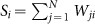, is homogeneous with a fast (exponentially) decaying tail (see Fig. 4). From a dynamical perspective, such a structure supports a high degree of local synchronization, although no global order is achieved, in agreement with observations in real systems. To better visualize the final topologies of the system in the different phases, in the right plots of Fig. 3 we have also reported the snapshots of networks typically produced in a single realization for the values of λ and T leading to the corresponding weight distributions. It is remarkable to notice how the modules in the networks on the left become more and more pronounced as λ increases, until the system breaks up into different components (λ = 0.3). Our results are consistent with many observed properties of the relationships between structure and dynamics during the formation of synchronized clusters51, indicating how network adaptation can actually be the mechanism at work in many real complex systems.

## Methods

### The network

The model has been implemented on weighted and directed random regular graphs, i.e. graphs where all the nodes have the same number of incoming and outgoing links, kin = koutk and the connections are purely random. The size of the graph is N = 300. For each simulation, we initially assign to each link of the network a constant weight Wij (t0) = 1/k. This ensures that the constraint(i = 1,…,N) is satisfied. We have checked that the results are almost independent of the connectivity k, provided that its value is sufficiently high (k ≥ 20). Hence, in all results presented here we fixed the initial connectivity at the value of k = 20.

### Numerical integration

Equations (1) and (2) are solved by a 4 th order Runge-Kutta algorithm with time-step h = 0.02, considering a uniform distribution of natural frequencies g(ω) in the interval [−1/2, 1/2] and choosing at random the initial phases θi (t0) in the range [−π, π]. The changes in topology from time t−1 to time t are quantified by measuring the quantity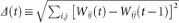. The system is considered to be in a stationary state when the condition Δ < 10−6 holds. The corresponding oscillators’ dynamics is characterized by the time-averages of the values of r(t) and rlink (t). The values reported in the figures for each value of λ and T are calculated as averages over 100 independent network realizations. Time is expressed in steps of Runge-Kutta algorithm.

### Order parameters

The original Kuramoto model assumes static and all-to-all interactions of equal strength. The key quantity to understand the dynamics of the system turns out to be a complex order parameter, which quantifies the extent of synchronization of the N oscillators40:The magnitude r(t) [0, 1] measures the phase coherence, while Ψ(t) is the average phase of the system. In particular, the value r = 1 describes a perfectly synchronized state, while for the incoherent solution r = 0. The long-time value of r, taken as a function of the coupling strength λ, displays a second order phase transition with a critical coupling λc = 2/πg(ω = 0), where g(ω) is the distribution of the natural frequencies ω, assumed to be uni-modal and even40. Besides, it has been numerically shown that r displays a second order phase transition also when a non-trivial interaction pattern is considered, with the critical coupling depending on the topological properties of the network7.

Apart from the global synchronization parameter (4), it is also interesting to consider the average degree of local (phase) synchronization between connected pairs of nodes, which can be quantified by the local order parameter introduced in13,14, slightly modified to take into account the weights of the links:where Γ i is the set of all the nodes pointing to i. Notice that, in spite of the resemblance with the definition of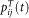in equation (3), rlink directly depends on this quantity only when the limit T → ∞ is considered. In particular, in this case rlink turns out to be proportional to the weighted average of all the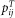. In our simulations we took Δt = 750 time units after checking that (for the distribution of internal frequencies {ωi } used in this work) a larger value of Δt does not change the values obtained for rlink .

### Community structures

As for the structural properties of the emerging networks in the stationary state, we focus on both their microscopic and mesoscopic description. Microscopically, we analyze the distribution of the link’s weights, P(Wij ) (i.e. the probability of finding a link with weight Wij ) and that of the nodes’s outgoing strength, P(Si ) (i.e. the probability of finding a node with outgoing strength). On the other hand, for the mesoscopic description, we focus on the modular structure of the networks. In particular, we apply a standard community detection algorithm: the extremal optimization of modularity, proposed by Duch and Arenas in52. The extremal optimization algorithm gives us a partition of the network into M non-overlapping communities or modules, so that each node of the network belongs to one community only. Once the modules of the network are found, we evaluate the modularity of the partition of the network by measuring its modular cohesion: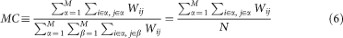Indexes α and β in formula stand for the modules, while i and j are node labels, as usual. The modular cohesion MC takes values in the range [0, 1] and is equal to 1 when the network is partitioned into non-interacting modules (separate components).